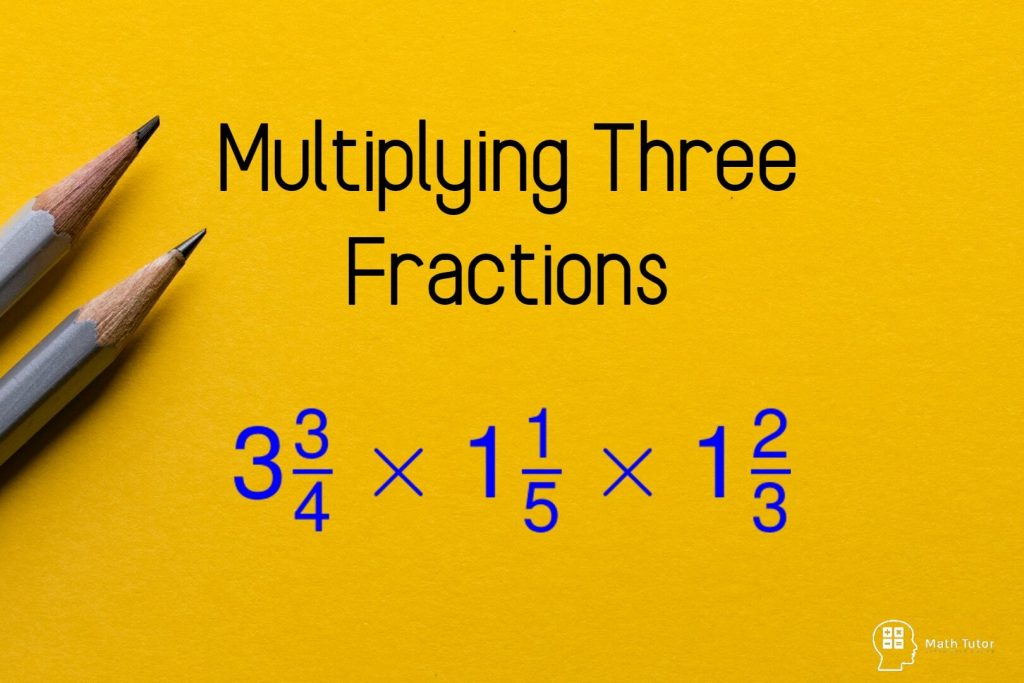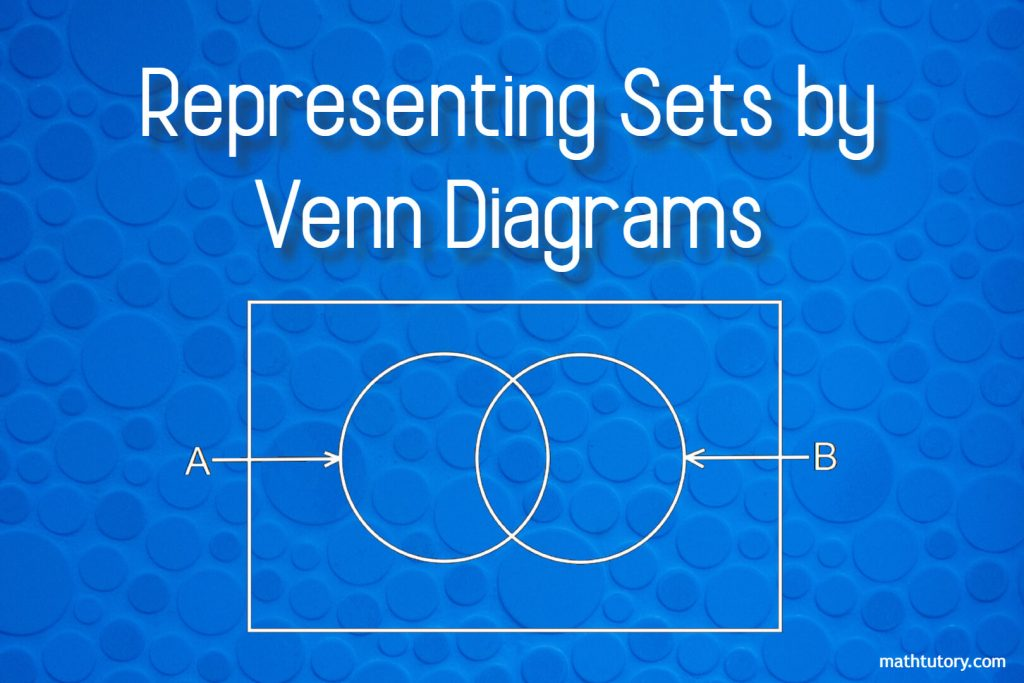# G. De Silva## Multiplying Three Fractions

In this lesson, we are going to learn how to multiplying three fractions.We have discussed how to multiply fractions from basics in another lesson. You can visit the following link to view the previous lesson.How to Multiply Fractions Multiplying Three Fractions Multiplying three fractions seems a little more complicated than multiplying two fractions. But the …## Representing Sets by Venn Diagrams

Venn diagrams are the most common way of representing a set. This method was introduced by the English mathematician John Venn. Therefore these are called Venn Diagram. Set is a collection of items that can be clearly identified.The items in a set are called its elements. Example: Let A be the set of even numbers …